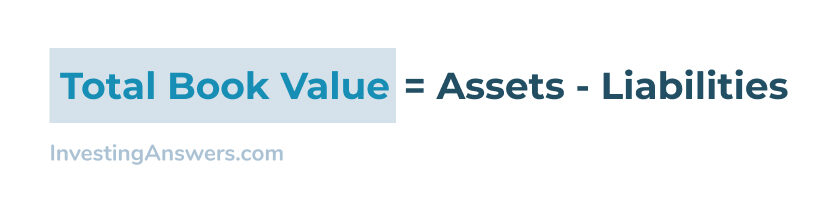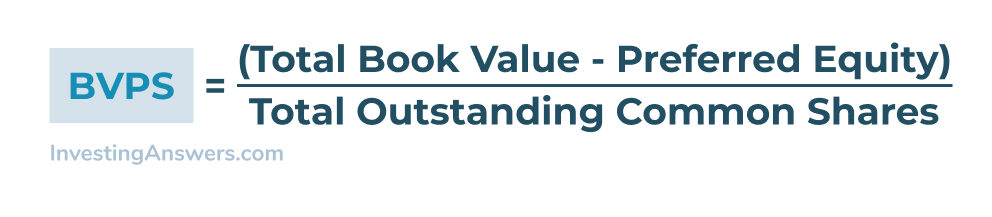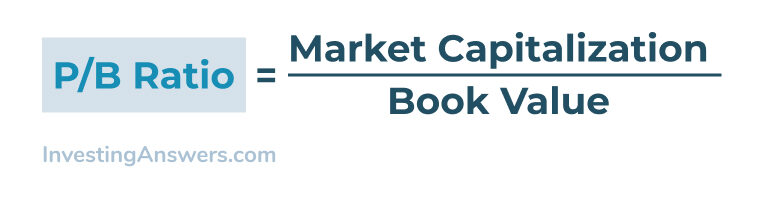Book value can be calculated using information from the balance sheet. Once you have that information you can also calculate the book value per share and is an indication as to whether or not a stock is over or undervalued.

This financial walkthrough not only details how to find book value and why it's important for investors.

## What is Book Value?

Book value refers to a company's net assets, calculated as the value of its assets net of (subtracting) its liabilities. It can also be calculated as the total shareholder equity of a company.

In practical terms, book value is the amount of equity a company has should it need to be liquidated (e.g. sell off assets to pay shareholders).

While the book value may show you the value of a company in terms of its assets, it is typically less than the market value that is reflected in the price of a share of its stock.

## How to Calculate Book Value (+ Example)

Book value can be expressed in two different ways:

• The total book value of a company

• The total book value per-share

Both of these numbers are simple to calculate and show investors how well a company is doing financially.

### How to Calculate Book Value From a Balance Sheet

Here is how you calculate the total book value of a company using its balance sheet numbers:This calculation is simple, but it shows exactly how much value a company has on the basis of its assets and debts.

### Book Value Calculation Example

Here's a simplified example of how to find the book value of a publicly-traded company.

In Q1 of 2021, Microsoft had a total asset value of \$301 billion and the total liability balance was \$177 billion.

\$301 billion minus \$177 billion = \$124 billion

This gives Microsoft an estimated book value of \$124 billion.

### How to Calculate Book Value per Share

Book value per share is calculated by looking at how much equity the company's assets provide per share of common stock that is issued. This helps give insight into how much would be paid out, per share, if a company were to liquidate its assets.

Here's the formula for how to calculate Book Value per Share:This formula takes the total book value, subtracts the preferred shareholder equity, and then divides by the total outstanding shares of common stock. This gives an exact book value price per share of common stock.

### Book Value per Share Example

Using the above example, here what the book value per share is for Microsoft:

In Q1 of 2021, Microsoft had a book value of \$124 billion and 7.56 billion outstanding shares of common stock.

Dividing the \$124 billion by the 7.56 billion outstanding shares = \$16.40 book value per share

The \$16.40 is how much a Microsoft common stock shareholder could expect to receive should Microsoft liquidate its assets and pay out to shareholders.

## What Is Price to Book Ratio?

The Price-to-Book Ratio (P/B Ratio) is the comparison of a company's market capitalization (or market value) to its book value. Here's how to calculate the P/B ratio:Taking Microsoft, for example, we can take the market cap of 1.89 trillion, and divide it by the \$124 billion book value, resulting in a P/B Ratio of 15.24.

A P/B ratio over 1.0 shows that the market values this company more than just the price of its balance sheet assets.

## What Does Book Value Tell You?

Book value gives the savvy investor insight into how a company is managing its debt, as well as the inherent value of its assets.

Looking at the P/B Ratio can also help tell you if a company is over- or under-valued in the marketplace. A company with a very high P/B ratio may be overvalued, while a company with a low (or negative) P/B ratio may be undervalued.

## Can Book Value Be Negative?

Book value can be a negative number if a company has more debt than assets on its balance sheet.

For example: As of Q1 2021, Hewlett-Packard Inc. has \$4.16 billion in assets and \$7.38 billion in debt. This gives it a book value of negative \$3.22 billion. This means that in the event of a liquidation, common shareholders would not receive any funds.

A negative book value can be a warning of a company taking on too much debt, and may be a more risky investment.

## Book Value vs Market Value

The book value of a company is the value of the assets on its balance sheet, minus liabilities. The market value of a company is the total market capitalization based on the price-per-share of total outstanding shares in the market.

While the book value gives investors an idea of what the company could pay out in the event of a liquidation, the market value is typically greater as it prices in a company's profitability and investors' expectations of its future growth.